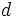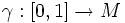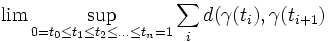# Minimizing geodesic

## Definition

### Given data

A metric space$M$ equipped with a metric$d$.

## Definition part

A path$\gamma: [0,1] \to M$ is termed a minimizing geodesic if it is the shortest path from$\gamma(0)$ to$\gamma(1)$.

By shortest, we mean path of minimum length where the length of a path is defined as:$\lim \sup_{0 = t_0 \le t_1 \le t_2 \le \ldots \le t_n = 1} \sum_i d(\gamma(t_i),\gamma(t_{i+1})$